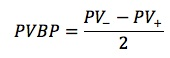#### Subject 5. Money Duration of a Bond and the Price Value of a Basis Point

Modified duration measures the percentage price change of a bond to a change in its yield-to-maturity. Money duration measures the absolute price change.

Money Duration = Dirty Price x Modified Duration

To calculate absolute price change:

ΔDirty Price = - Money Duration x ΔYield

In the U.S., money duration is called dollar duration. It is the approximate dollar change in a bond's price for a 100 basis point change in yield.

The price value of a basis point (PVBP) is the absolute change in the price of a bond for a one basis point change in yield. It is simply the money duration of a bond for a one basis point change in yield.

Example

Scott Marsh from Mass Avenue Research Management purchased a bond for a price of 93.555. This bond has a coupon of 14.70% and a modified duration of 3.00. Given market interest rates of 10.00% and a change in market rates of -66 basis points, what is the price value of a basis point?

The answer is the modified duration x 1 basis point x bond price or 3.00 x .0001 x 93.555 = \$0.0281

Note: The other information has been placed into this question as a distraction. Don't be fooled by extraneous data!

To calculate PVBP:P- is the full price calculated by lowering the yield-to-maturity by one basis point.
P+ is the full price calculated by raising the yield-to-maturity by one basis point.